# Probability Distributions and Random Variables

Last updated: January 13th, 2020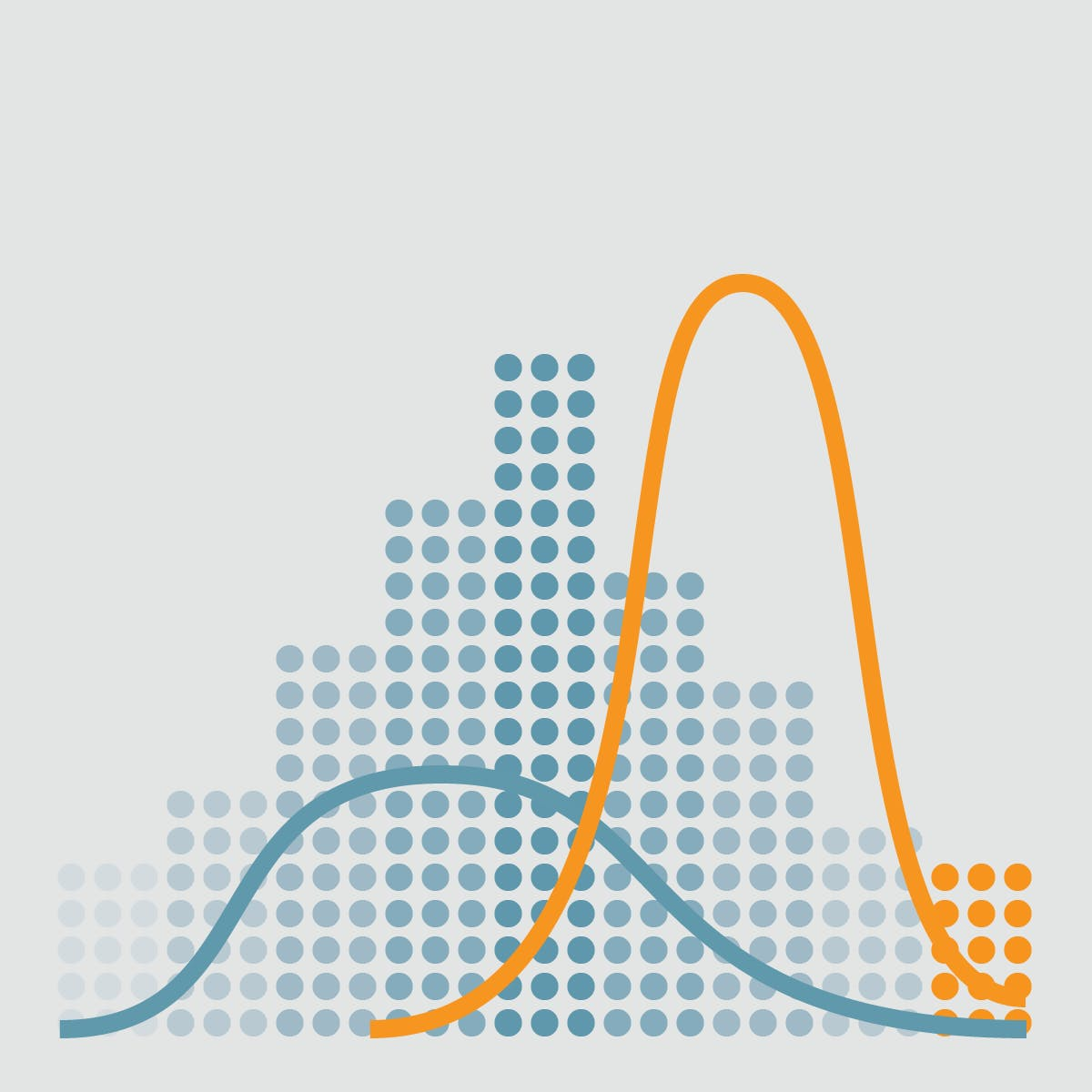# Probability Distributions and Random variables¶

In probability theory and statistics, a probability distribution is a mathematical function that provides the probabilities of occurrence of different possible outcomes in an experiment.## Hands on!¶

In :
import numpy as np
import pandas as pd
import matplotlib.pyplot as plt
import seaborn as sns

%matplotlib inline## Random variables¶

A random variable is a variable whose possible values are numerical outcomes of a random phenomenon. There are two types of random variables: discrete and continuous.

• Probability distribution of a discrete random variable is applicable to the scenarios where the set of possible outcomes is discrete (such as a coin toss or a roll of dice) and can be encoded by a discrete list of the probabilities of the outcomes, known as a probability mass.

Some examples of discrete probability distributions are Bernoulli distribution, Binomial distribution and Poisson distribution.

• Probability distribution of a continuous random variable, known as probability distribution functions, is applicable to the scenarios where the set of possible outcomes can take on values in a continuous range (e.g. real numbers), such as the temperature on a given day) is typically described by probability density functions (with the probability of any individual outcome actually being 0).

Some examples of continuous probability distributions are Uniform distribution, Normal distribution, Exponential distribution and Beta distribution.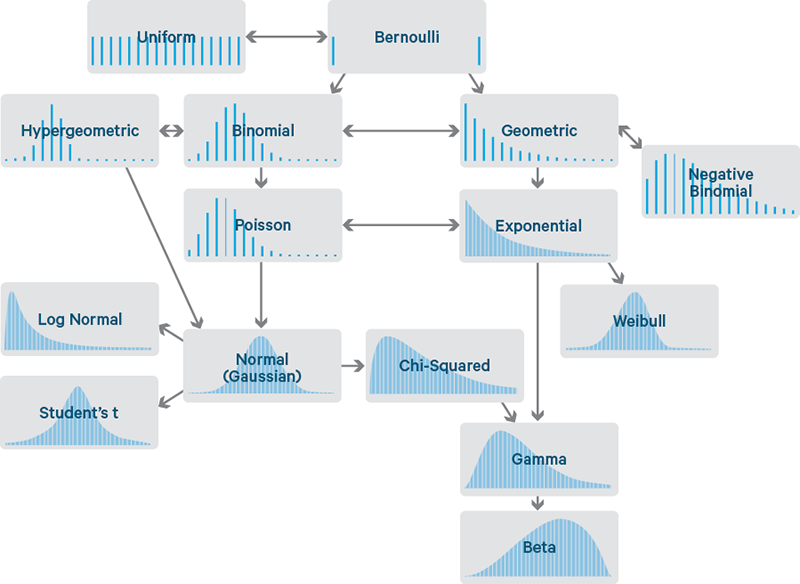We'll explain some of them:

• Continuous:
• Normal distribution.
• Uniform distribution.
• Gamma distribution.
• Exponential distribution.
• Discrete:
• Binomial distribution.
• Poisson distribution.## Modality¶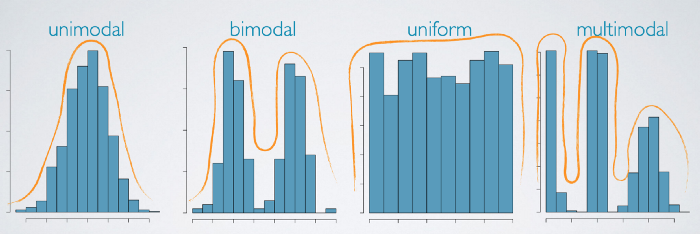A distribution might be unimodal with one prominent peak, bimodal with two prominent peaks, or uniform with no prominent peaks.

With more than two prominent peaks a distribution is usually said to be multimodal.

A bimodal distribution might indicate that there are two distinct groups in your data.

A distribution of heights of individuals at a preschool. The first peak might be the kids and the second might be the teachers.

A uniform distribution means there's no apparent trend in the data. That all values of the variable are equally likely to occur.

A distribution of date of birth (only month) show no trend as just as likely to be born in any month.## Normal distribution¶

Normal Distribution, also known as Gaussian distribution, is probably the most common distribution. You will encounter it at many places especially in topics of statistical inference. It is one of the assumptions of many data science algorithms too.

A normal distribution has a bell-shaped density curve described by its mean $\mu$ and standard deviation $\sigma$. The density curve is symmetrical, centered about its mean, with its spread determined by its standard deviation showing that data near the mean are more frequent in occurrence than data far from the mean.

The probability distribution function of a normal density curve with mean $\mu$ and standard deviation $\sigma$ at a given point $x$ is given by:

$$f(x|\mu, \sigma^2) = \frac{1}{\sqrt{2\pi\sigma^2}}e^{-\frac{(x-\mu)^2}{2\sigma^2}}$$
In :
normal = pd.DataFrame()
normal['x'] = np.random.normal(0, 1, 10000) # loc, scale, size
normal['y'] = np.random.normal(5, 1, 10000)
normal['z'] = normal['x'] + normal['y']

plt.figure(figsize=(14,6))

sns.distplot(normal['z'])

# Mean line
plt.axvline(normal['z'].mean(), color='#e74c3c', linestyle='dashed', linewidth=2)

Out:
<matplotlib.lines.Line2D at 0x7fab8bd63a20>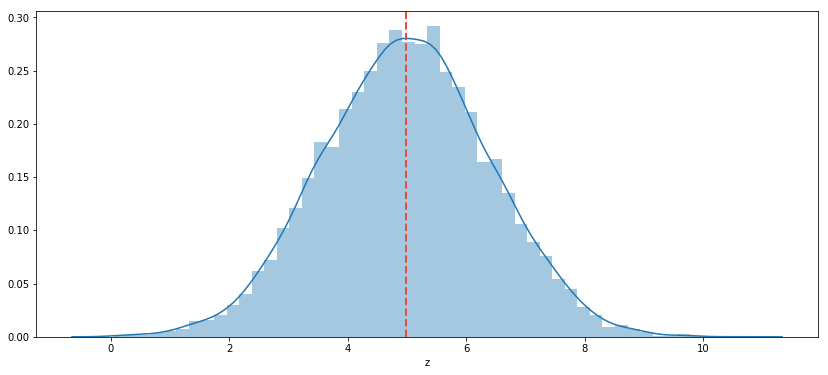### Real life examples¶

• Show size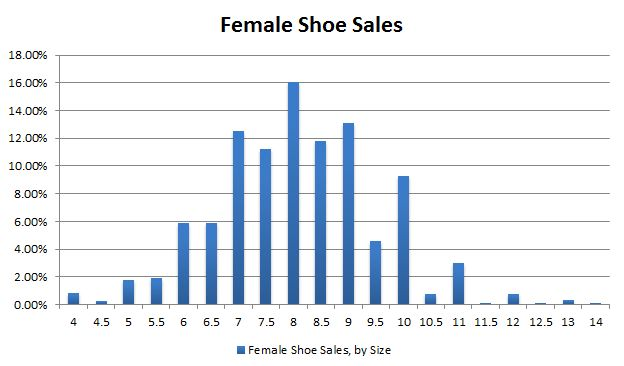• Birth weight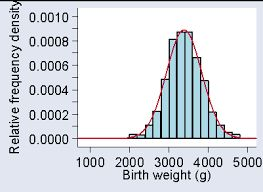- Income Distribution in Economy

- Student's Average report## Uniform distribution¶

The probability distribution function of the continuous uniform distribution is:

$$\left\{\begin{matrix} \frac{1}{b-a} & for\ a \leq x \leq b, \\ 0 & for\ x < a\ or\ x > b \end{matrix}\right.$$

Since any interval of numbers of equal width has an equal probability of being observed, the curve describing the distribution is a rectangle, with constant height across the interval and 0 height elsewhere.

In :
uniform = np.random.uniform(1, 50, 10000) # low, high, size

plt.figure(figsize=(14,6))

sns.distplot(uniform)

# Mean line
plt.axvline(uniform.mean(), color='#e74c3c', linestyle='dashed', linewidth=2)

Out:
<matplotlib.lines.Line2D at 0x7fab89a57cf8>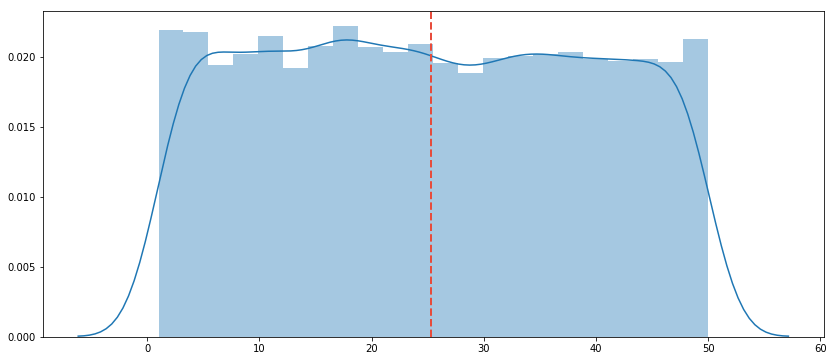### Real life examples¶

This is the theoretical distribution model for:

• Balanced coin
• An unbiased die
• A casino roulette.
• The first card of a well-shuffled deck.## Gamma distribution¶

The gamma distribution is a two-parameter family of continuous probability distributions.

While it is used rarely in its raw form but other popularly used distributions like exponential, chi-squared, erlang distributions are special cases of the gamma distribution.

In :
gamma = np.random.gamma(2, 200, 10000) # shape, scale, size

plt.figure(figsize=(14,6))

sns.distplot(gamma)

# Mean line
plt.axvline(gamma.mean(), color='#e74c3c', linestyle='dashed', linewidth=2)

Out:
<matplotlib.lines.Line2D at 0x7fab89baed30>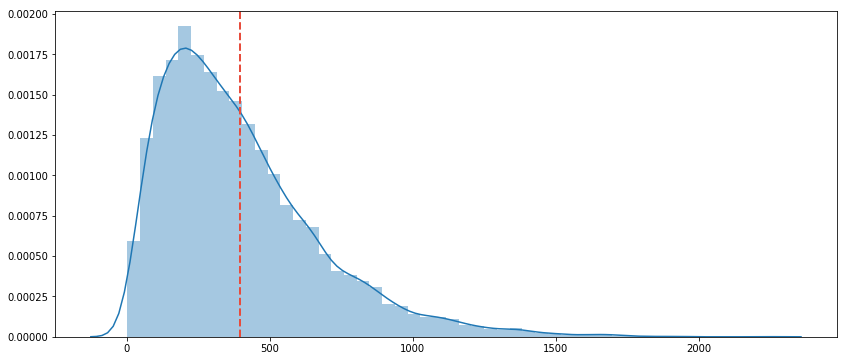### Real life examples¶

• Time-to failure of a machinery or device in an industrial context.
• In finance and insurance, it is commonly used as a model for financial losses.
• Insurance claim sizes.
• It is also commonly used to model waiting times.## Exponential distribution¶

The exponential distribution describes the time between events in a Poisson point process, i.e., a process in which events occur continuously and independently at a constant average rate. It has a parameter $\lambda$ called rate parameter, and its equation is described as:

$$f(x, \lambda) = \left\{\begin{matrix} \lambda e^{-\lambda x} & for\ x \geq 0, \\ 0 & for\ x < 0 \end{matrix}\right.$$
In :
exponential = np.random.exponential(0.5, 10000) # a, b, size

plt.figure(figsize=(14,6))

sns.distplot(exponential)

# Mean line
plt.axvline(exponential.mean(), color='#e74c3c', linestyle='dashed', linewidth=2)

Out:
<matplotlib.lines.Line2D at 0x7fab89a6c8d0>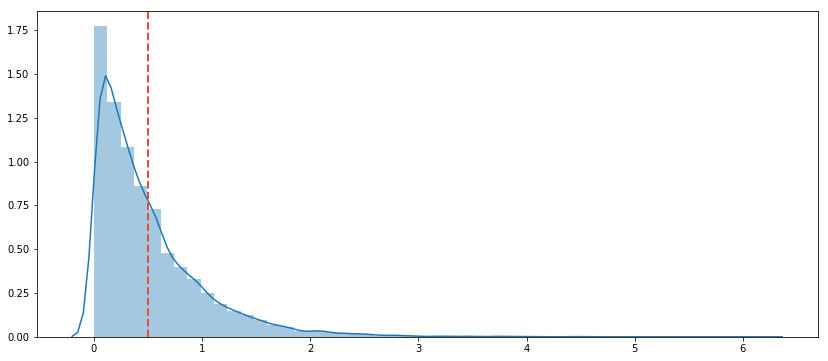### Real life examples¶

• Time between 911 calls during peak hours.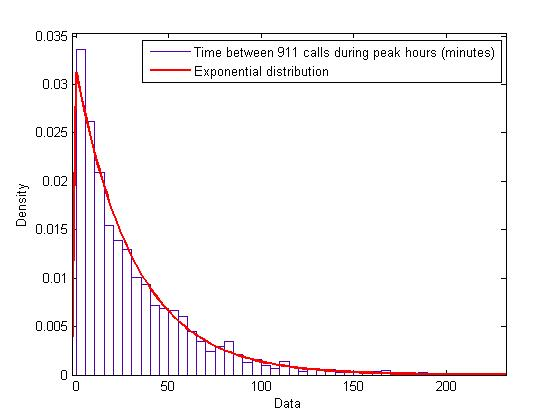• Customers attention time.
• Testing product reliability.
• Building continuous-time Markov chains.## Binomial distribution¶

Binomial is a discrete probability distribution of the number of successes in a sequence of $n$ independent yes/no experiments, such as success or failure, gain or loss, win or lose, each of which yields success with probability p.

The parameters of a binomial distribution are $n$ and $p$ where $n$ is the total number of trials, and $p$ is the probability of success in each trial. Its probability distribution function is given by:

$$f(k,n,p) = Pr(k,n,p) = Pr(X=k) = \begin{pmatrix} n\\ k \end{pmatrix} p^k (1-p)^{n-k}$$

where:

$$\begin{pmatrix} n\\ k \end{pmatrix} = \frac{n!}{k!(n-k)!}$$
In :
binomial = np.random.binomial(100, 0.5, 10000) # n, p, size

print('Mean: %s' % binomial.mean())

plt.figure(figsize=(14,6))

sns.countplot(binomial)

Mean: 49.9887

Out:
<matplotlib.axes._subplots.AxesSubplot at 0x7fab89abb160>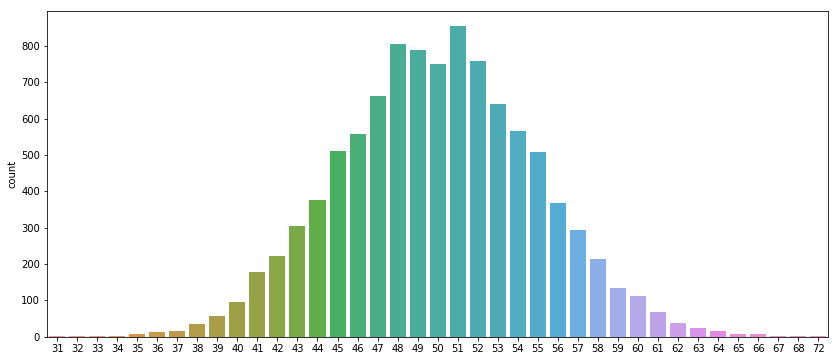### Real life examples¶

• Tossing a coin 20 times to see how many tails occur.
• Asking 200 people if they watch ABC news.
• The probability that Susan will beat Shannon in two of their three tennis matches.
• The probability of getting a full house poker hand.
• The probability that all 5 of your randomly-chosen group members will have passed the midterm.
• The probability that a student blindly guessing will get at least 8 out of 10 multiple-choice questions correct.

### Examples which aren't binomial experiments¶

• Rolling a die until a 6 appears (not a fixed number of trials).
• Asking 20 people how old they are (not two outcomes).
• Drawing 5 cards from a deck for a poker hand (done without replacement, so not independent).## Poisson distribution¶

Poisson is a discrete probability distribution that expresses the probability of a given number of events occurring in a fixed interval of time and/or space if these events occur with a known average rate and independently of the time since the last event.

Poisson distribution is described in terms of the rate ($\mu$) at which the events happen. An event can occur 0, 1, 2, … times in an interval. The average number of events in an interval is designated $\lambda$ (lambda). Lambda is the event rate, also called the rate parameter. The probability of observing $k$ events in an interval is given by the equation:

$$P(k\ events\ in\ interval)=e^{-\lambda} \frac{\lambda^k}{k!}$$
In :
poisson = np.random.poisson(15, 10000) # lam, size

print('Mean: %s' % poisson.mean())

plt.figure(figsize=(14,6))

sns.countplot(poisson)

Mean: 15.0688

Out:
<matplotlib.axes._subplots.AxesSubplot at 0x7fab89849160>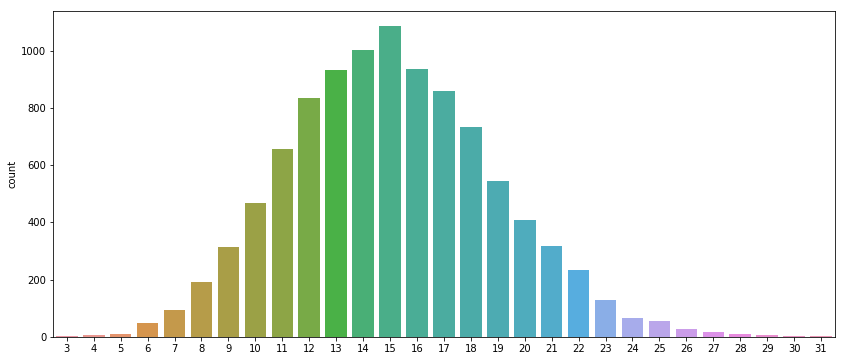### Real life examples¶

The Poisson process gives you a way to find probabilities for random points in time for a process. A 'process' could be almost anything:

• Accidents at an interchange.
• File requests on a server.
• Customers arriving at a store.
• Battery failure and replacement.